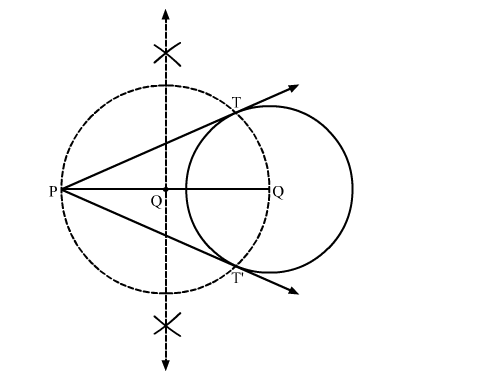# Draw a circle of radius 3 cm. From a point P, 7 cm away from the centre of the circle,`
Question:

Draw a circle of radius 3 cm. From a point P, 7 cm away from the centre of the circle, draw two tangents to the circle. Also, measure the lengths of the tangents.

Solution:

Steps of Construction

Step 1. Draw a circle with O as centre and radius 3 cm.

Step 2. Mark a point P outside the circle such that OP = 7 cm.

Step 3. Join OP. Draw the perpendicular bisector XY of OP, cutting OP at Q.

Step 4. Draw a circle with Q as centre and radius PQ (or OQ), to intersect the given circle at the points T and T'.

Step 5. Join PT and PT'.Here, PT and PT' are the required tangents.

PT = PT' = 6.3 cm (Approx)